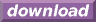#Calcute: freeware calculator

## Square(sqr)

The square of a value is this value raised to the power of 2 (raised to the second power). Calcute implements this operation with the sqr function. The same result will be obtained using the power operator ^ with the value of 2, or by multiplying the number by itself: sqr(x) = x^2 = x*x.

```sqr(3)
9
3^2
9
3*3
9
```